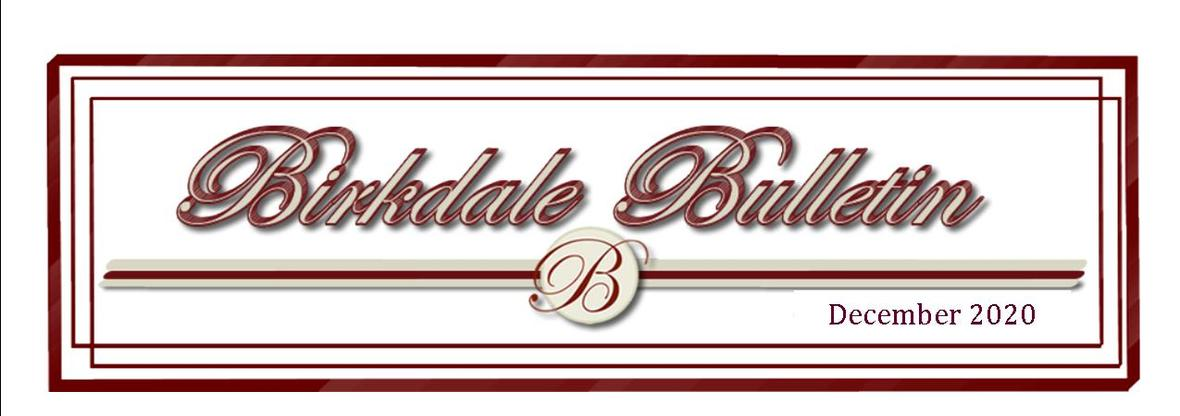President's Message- December 2020

 table div table+table+table+table+table div table{width:100%;padding:0}table div table+table+table+table+table div table img{width:96.23%;padding:0;float:none}table div table+table+table+table+table div table td{width:100%;padding:0 1.88% 18px}/* styles */Treasurer's Report- December

 table div table+table+table+table+table+table+table+table+table div table{width:100%;padding:0}table div table+table+table+table+table+table+table+table+table div table img{width:96.23%;padding:0;float:none}table div table+table+table+table+table+table+table+table+table div table td{width:100%;padding:0 1.88% 18px}/* styles */Birkdale's Most Recent Financial Statement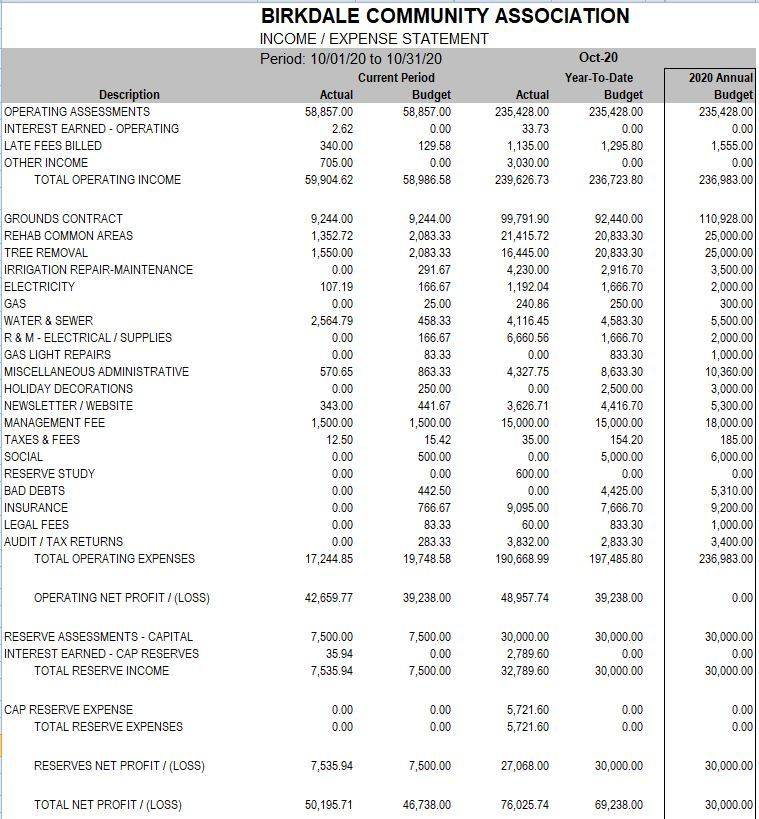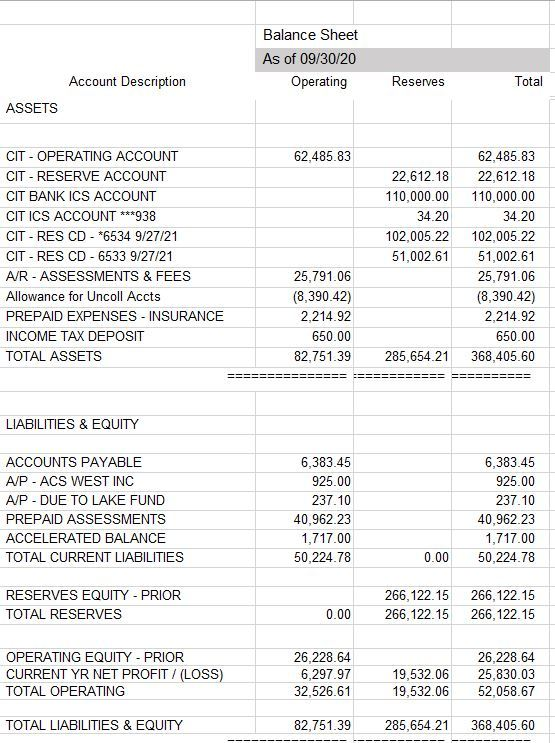table div table+table+table+table+table+table+table+table+table+table+table+table+table div table{width:100%;padding:0}table div table+table+table+table+table+table+table+table+table+table+table+table+table div table img{width:96.23%;padding:0;float:none}table div table+table+table+table+table+table+table+table+table+table+table+table+table div table td{width:100%;padding:0 1.88% 18px}/* styles */ACS West is our Managing Agent

You may use your coupon book to send in your payment or set up quarterly payments through ACS West or your bank.

REMINDER: Next assessment is due January 1st.

Thank you!

 table div table+table+table+table+table+table+table+table+table+table+table+table+table+table+table div table{width:100%;padding:0}table div table+table+table+table+table+table+table+table+table+table+table+table+table+table+table div table img{width:96.23%;padding:0;float:none}table div table+table+table+table+table+table+table+table+table+table+table+table+table+table+table div table td{width:100%;padding:0 1.88% 18px}/* styles */News from the Architectural Review CommitteeDecember is typically a busy holiday month. I have already seen many of our neighbors decorating for the Christmas holiday. I guess we all are looking for a little cheer during these trying times. One thing we still need to remember is to make sure we are practicing social distancing during this pandemic. Also, we need to remember to wear face masks when visiting neighbors. We can only hope that this will help to keep the virus from spreading.
The ARC Committee would like to wish everyone a Happy Holiday. Remember to be safe.

Applications submitted to ARC for approval:

8843 Torrey Pines Drive -Removal of a tree
9049 Spyglass Hill Turn - Patio
9048 Spyglass Hill Turn - Roof
9048 Spyglass Hill Turn - Removal of 4 trees

Happy Holidays from the ARC.

Lee Kemmet
ARC Committee

Mailbox Maintenance

Mailbox maintenance is the homeowner’s responsibility, and the specific requirements are in the ARC Guidelines.

When painting your mailbox, Lowe’s on Hull Street has the equivalent paint color as Benjamin Moore which is Lowe's - Valspar, Quart, 372, Birkdale Vanilla Cookie, Exterior Satin, Duramax, 105-1, 107-1, 214-2.5.

For your metal mailbox, Cottage Red Benjamin Moore is available at Virginia Paint and can also be mixed at Lowes.

The Birkdale logo and numbers may be purchased from Sign Crafters Inc., Attn: Barbara Isenberg, 804-379-2004 or email bisenberg@sign-crafters.com

OR

You may contact resident Rich McGinness - The Mailbox Guy- who will assist you with mailbox maintenance.

Rich McGuinness at Themailboxguy@yahoo.com or call 919-621-9983

 table div table+table+table+table+table+table+table+table+table+table+table+table+table+table+table+table+table+table div table{width:100%;padding:0}table div table+table+table+table+table+table+table+table+table+table+table+table+table+table+table+table+table+table div table img{width:96.23%;padding:0;float:none}table div table+table+table+table+table+table+table+table+table+table+table+table+table+table+table+table+table+table div table td{width:100%;padding:0 1.88% 18px}/* styles */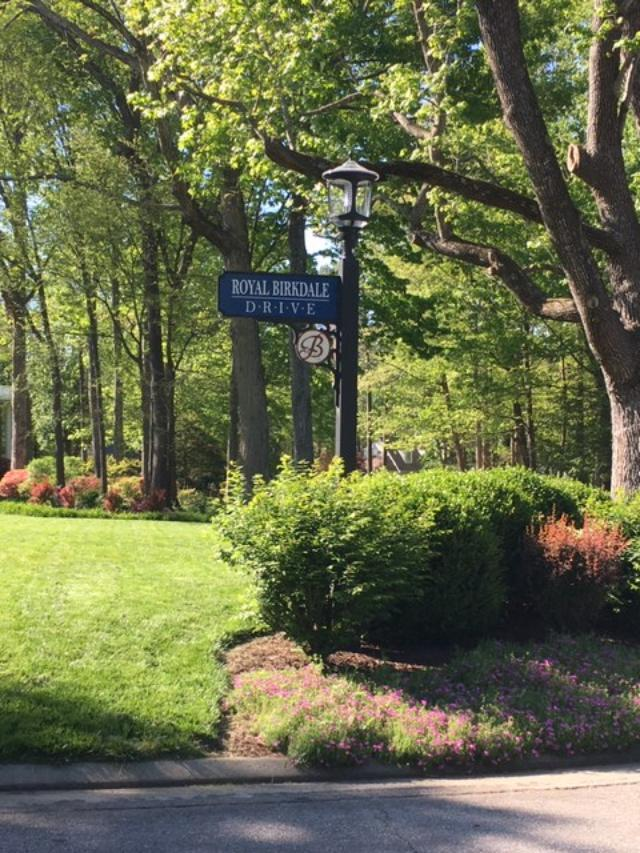Landscape & Neighborhood Etiquette

NO DUMPING- Please do not drag brush and clippings or debris into the common areas to include the area surrounding the power lines behind Royal Birkdale. Those areas belong to you and to me as property owners.

PARKING- Do not park on the green spaces in Birkdale. Please park in your driveway rather than on the street fronting your home. Street parking limits access to emergency vehicles, causes congestion, and is a hazard for children and walkers.

NO FIRES whatsoever. Fires require a permit from Chesterfield County. It is not appropriate to burn any vegetation in common areas and cause alarm to nearby homeowners.

TRASH- Birkdale has a backyard trash policy. Please do not take you trash cans to the curb. There are many companies who offer backyard pickup services in our neighborhood.

DOG WASTE- Please be courteous and pick up after your pooch. While walking please make an effort to keep your dog from using the bathroom on other resident's property. Direct them to the mulched common areas whenever possible-and pick up!

If you have any problems or complaints regarding common areas, please call Wayne Bass at 804-639-0795.

Lighting

Gas street lights were replaced with solar powered LED lights last year and are now the standard from the Winterpock Road entrance to the Spring Run Road entrance. Solar power is dependent on adequate sunlight to recharge the batteries. Thus in winter, lights may not function all night. If you have questions or notice a situation which may need attention, call ACS West for investigation.

 table div table+table+table+table+table+table+table+table+table+table+table+table+table+table+table+table+table+table+table+table div table{width:100%;padding:0}table div table+table+table+table+table+table+table+table+table+table+table+table+table+table+table+table+table+table+table+table div table img{width:96.23%;padding:0;float:none}table div table+table+table+table+table+table+table+table+table+table+table+table+table+table+table+table+table+table+table+table div table td{width:100%;padding:0 1.88% 18px}/* styles */Covenants Committee

This report will address several issues which are common or anticipated in Birkdale. Homeowners are expected to be aware of and comply with the ARC Standards and Guidelines which are part of the Association Governing Documents. This document can be found on the Birkdale website.

SIGNS – There are several political signs and some from graduation celebrations remaining. Political signs should be removed no later than 3 days following the election. (Ref ARC Guidelines 6.32 B) The graduation celebratory signs were a special application and should have been removed.

HOLIDAY DECORATIONS – ARC Guidelines 6.21 D addresses the installation and removed of holiday lighting. ARC approval is not required for installation of holiday or festive lighting. Lighting must coincide with the holiday and must be removed within 14 days of the holiday, i.e. January 7th.

SIDING MILDEW – Several houses exhibit mildew or other stains, generally on the north side of the house. These need to be power washed to correct the violation. (Ref ARC Guidelines, 7.01 B.e)

TRASH CANS – In accordance with ARC standards 7.02, no curbside trash pick-up is permitted. New residents especially seem to be unaware of this requirement. There are several contactors serving Birkdale which comply with this.

LANDSCAPING – Winter is not the most active period for landscaping, but it is a good time for planning. Pruning and trimming can be done. Leaf and broken limb removal are also normal winter activities. If irrigation systems have not been winterized, this needs to be done.

 table div table+table+table+table+table+table+table+table+table+table+table+table+table+table+table+table+table+table+table+table+table+table div table{width:100%;padding:0}table div table+table+table+table+table+table+table+table+table+table+table+table+table+table+table+table+table+table+table+table+table+table div table img{width:96.23%;padding:0;float:none}table div table+table+table+table+table+table+table+table+table+table+table+table+table+table+table+table+table+table+table+table+table+table div table td{width:100%;padding:0 1.88% 18px}/* styles */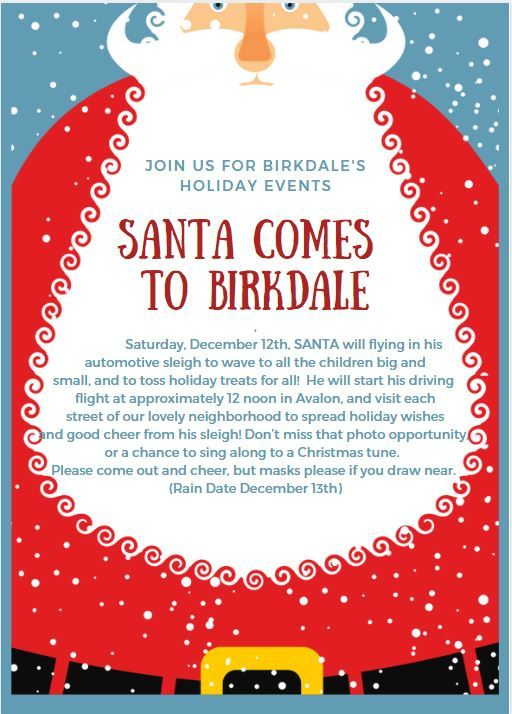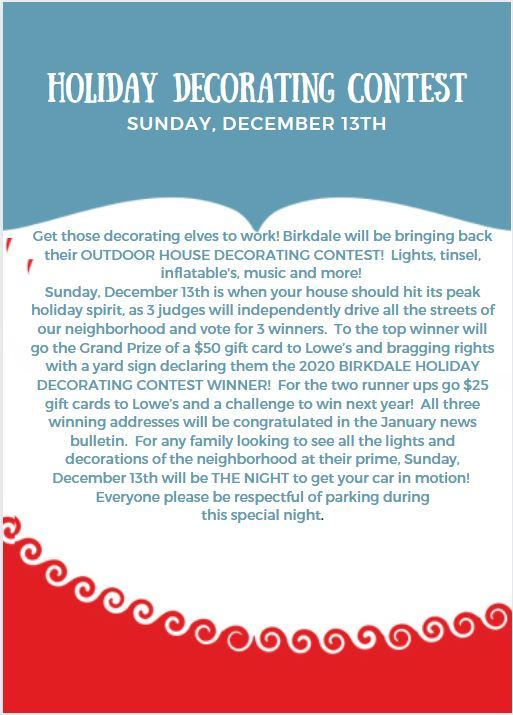table div table+table+table+table+table+table+table+table+table+table+table+table+table+table+table+table+table+table+table+table+table+table+table+table+table div table{width:100%;padding:0}table div table+table+table+table+table+table+table+table+table+table+table+table+table+table+table+table+table+table+table+table+table+table+table+table+table div table img{width:96.23%;padding:0;float:none}table div table+table+table+table+table+table+table+table+table+table+table+table+table+table+table+table+table+table+table+table+table+table+table+table+table div table td{width:100%;padding:0 1.88% 18px}/* styles */Birkdale's Pet of the Month

Meet Nova-a sweet German Shepherd who loves kids, people and other dogs. She is owned by the Phillips family and lives on Easton Ridge Place. The Phillips moved to Birkdale in May 2020 but haven’t been able to meet as many people as they would have liked because of Covid. However, after getting Nova more people have stopped to say hello. ❤️ Love that a sweet dog can help neighbors connect!

Would you like to see your pet featured in the Bulletin?

Send us a photo of your pet and a brief description to appear as next month's Pet of the Month

email to:
Subject line- Pet of the Month

 table div table+table+table+table+table+table+table+table+table+table+table+table+table+table+table+table+table+table+table+table+table+table+table+table+table+table+table div table{width:100%;padding:0}table div table+table+table+table+table+table+table+table+table+table+table+table+table+table+table+table+table+table+table+table+table+table+table+table+table+table+table div table img{width:96.23%;padding:0;float:none}table div table+table+table+table+table+table+table+table+table+table+table+table+table+table+table+table+table+table+table+table+table+table+table+table+table+table+table div table td{width:100%;padding:0 1.88% 18px}/* styles */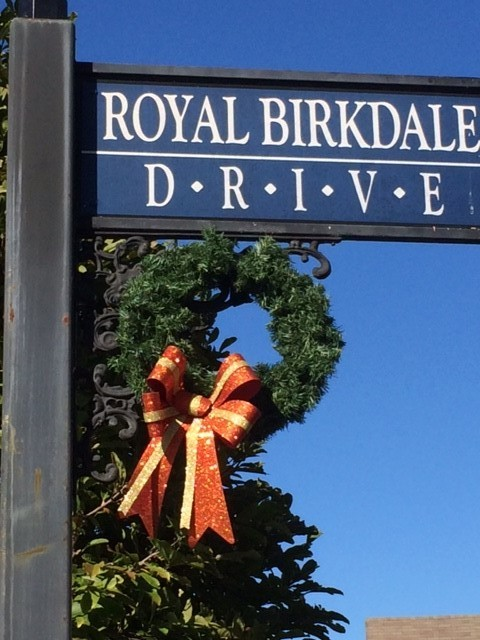THANK YOU TO OUR HOLIDAY DECORATORS-

This year we would like to thank the following "2020 Wreath Volunteers" for coming out and donating their time to make Birkdale look festive for the holidays.

Baugh, Bishop, Calhoun, Haring, Lasorsa, Lackey
Lowe, Maxwell, Moore, Nevel, Oliver, Sugumele
Wallace

A Special Thank You to Betsy and Nick Martello for their many year of volunteering with organizing the hanging of the wreaths.

Christine Nevel
Csquared122@verizon.net
804-852-8949

 table div table+table+table+table+table+table+table+table+table+table+table+table+table+table+table+table+table+table+table+table+table+table+table+table+table+table+table+table+table div table{width:100%;padding:0}table div table+table+table+table+table+table+table+table+table+table+table+table+table+table+table+table+table+table+table+table+table+table+table+table+table+table+table+table+table div table img{width:96.23%;padding:0;float:none}table div table+table+table+table+table+table+table+table+table+table+table+table+table+table+table+table+table+table+table+table+table+table+table+table+table+table+table+table+table div table td{width:100%;padding:0 1.88% 18px}/* styles */Chesterfield Students Resume an all Virtual Schedule Through the end of January.

With Covid cases exceeding 25 in 100,000 cases, Chesterfield County Schools resuming virtual classes. For information on schedules and updates please visit the county website at https://mychesterfieldschools.com/

Please continue to slow down when driving through the neighborhood as children will be out riding bikes and playing. Please avoid parking in the streets as it blocks the view and affects the safety of everyone.

Winter Break is December 21st-January 1st. Here is the full 2020-2021 School Calendar.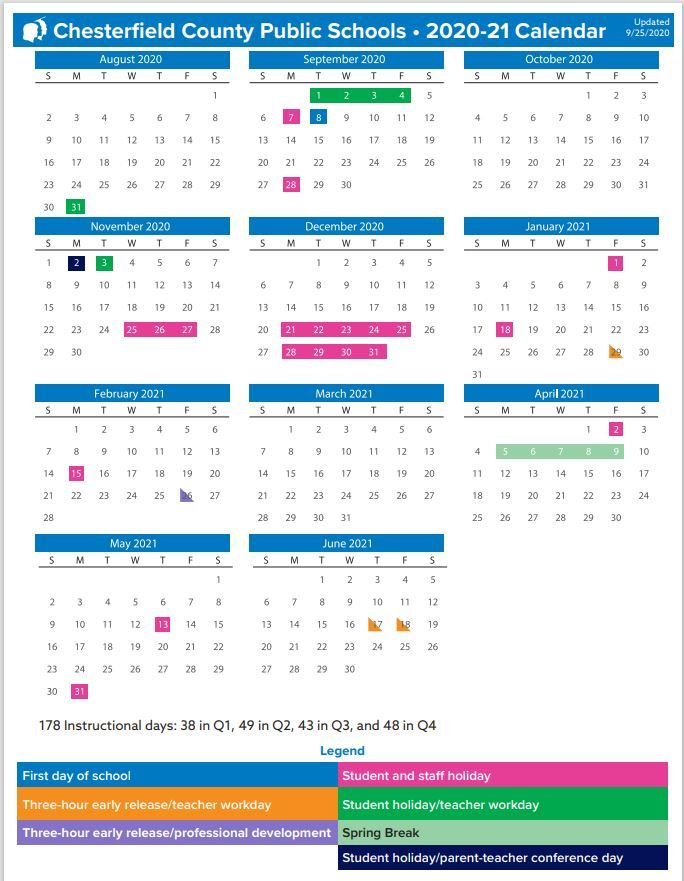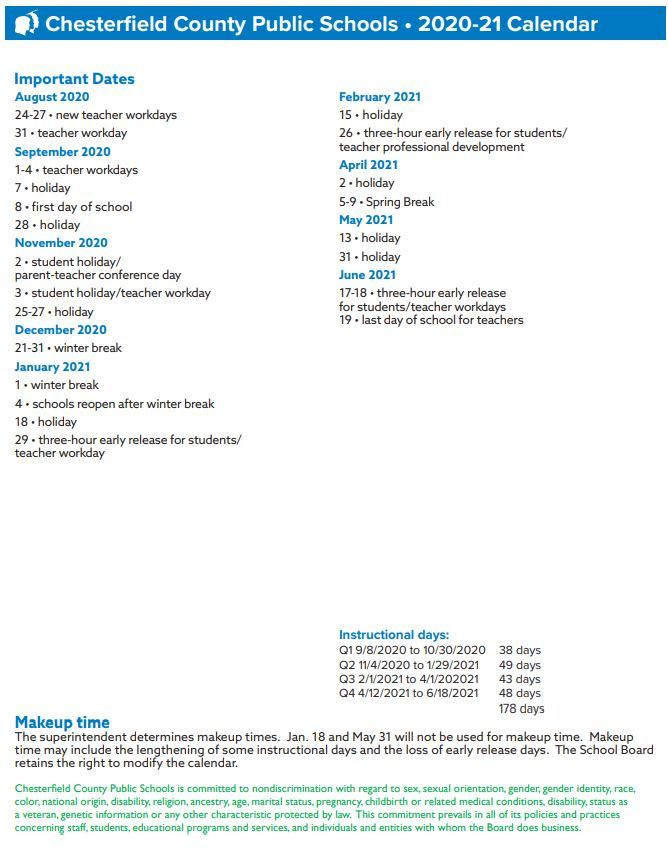table div table+table+table+table+table+table+table+table+table+table+table+table+table+table+table+table+table+table+table+table+table+table+table+table+table+table+table+table+table+table+table+table+table div table{width:100%;padding:0}table div table+table+table+table+table+table+table+table+table+table+table+table+table+table+table+table+table+table+table+table+table+table+table+table+table+table+table+table+table+table+table+table+table div table img{width:96.23%;padding:0;float:none}table div table+table+table+table+table+table+table+table+table+table+table+table+table+table+table+table+table+table+table+table+table+table+table+table+table+table+table+table+table+table+table+table+table div table td{width:100%;padding:0 1.88% 18px}/* styles */Neighborhood Watch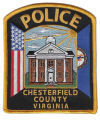Any suspicious activity should always be reported to the local police to investigate.

Bear and fox sightings should be reported to the Animal Control number.

Emergency 911 | Non-emergency 804-748-1251

Animal Control 748-1683

Traffic Hotline 804-318-8084 or speeding@chesterfield.gov

 table div table+table+table+table+table+table+table+table+table+table+table+table+table+table+table+table+table+table+table+table+table+table+table+table+table+table+table+table+table+table+table+table+table+table+table+table div table{width:100%;padding:0}table div table+table+table+table+table+table+table+table+table+table+table+table+table+table+table+table+table+table+table+table+table+table+table+table+table+table+table+table+table+table+table+table+table+table+table+table div table img{width:96.23%;padding:0;float:none}table div table+table+table+table+table+table+table+table+table+table+table+table+table+table+table+table+table+table+table+table+table+table+table+table+table+table+table+table+table+table+table+table+table+table+table+table div table td{width:100%;padding:0 1.88% 18px}/* styles */2021 BIRKDALE PROPOSED COMMUNITY ASSOCIATION CALENDAR

*The calendar will be finalized at the first 2021 Board Meeting

February 4th- Board Meeting

May 6th - Board Meeting

May 8th- Semi-Annual Spring Yard Sale

August 5th- Board Meeting

September 9th-Board Budget Meeting

October 2nd- Semi-Annual Fall Yard Sale

October 7th- Annual Board Meeting

October 16th- Fall Festival

November 6th- Board Meeting

 table div table+table+table+table+table+table+table+table+table+table+table+table+table+table+table+table+table+table+table+table+table+table+table+table+table+table+table+table+table+table+table+table+table+table+table+table+table+table div table{width:100%;padding:0}table div table+table+table+table+table+table+table+table+table+table+table+table+table+table+table+table+table+table+table+table+table+table+table+table+table+table+table+table+table+table+table+table+table+table+table+table+table+table div table img{width:96.23%;padding:0;float:none}table div table+table+table+table+table+table+table+table+table+table+table+table+table+table+table+table+table+table+table+table+table+table+table+table+table+table+table+table+table+table+table+table+table+table+table+table+table+table div table td{width:100%;padding:0 1.88% 18px}/* styles */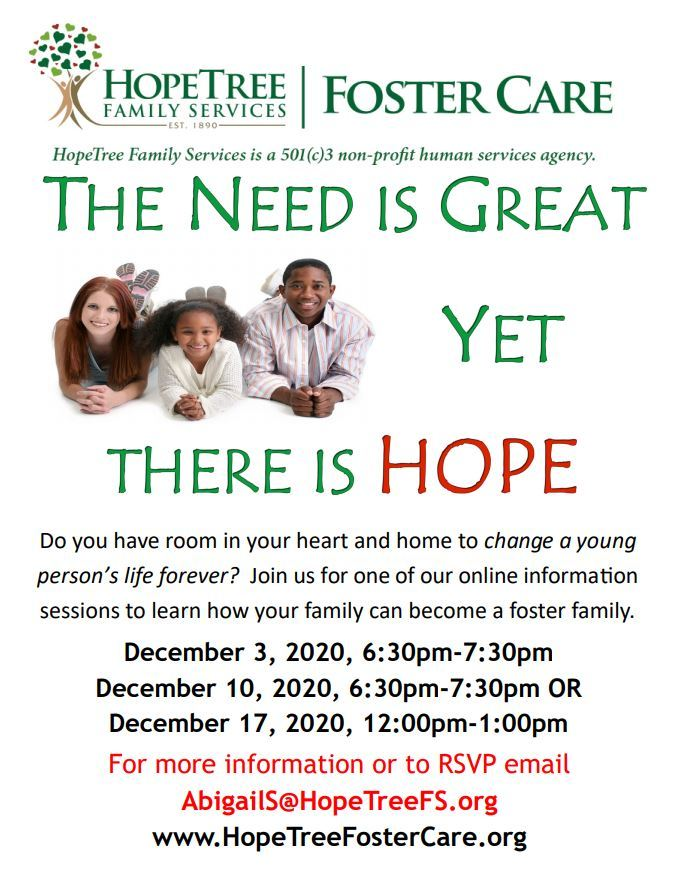table div table+table+table+table+table+table+table+table+table+table+table+table+table+table+table+table+table+table+table+table+table+table+table+table+table+table+table+table+table+table+table+table+table+table+table+table+table+table+table+table div table{width:100%;padding:0}table div table+table+table+table+table+table+table+table+table+table+table+table+table+table+table+table+table+table+table+table+table+table+table+table+table+table+table+table+table+table+table+table+table+table+table+table+table+table+table+table div table img{width:96.23%;padding:0;float:none}table div table+table+table+table+table+table+table+table+table+table+table+table+table+table+table+table+table+table+table+table+table+table+table+table+table+table+table+table+table+table+table+table+table+table+table+table+table+table+table+table div table td{width:100%;padding:0 1.88% 18px}/* styles */Want to participate on a committee? This is a great way to meet and work with neighbors for a common goal: helping our community. We are currently looking for Neighborhood Watch, Covenants and Social Committee chairs.

Please send a message to this newsletter with your interests or contact any Board member, and the BCA will gladly and willingly find a place to use your talents! Many thanks!

 table div table+table+table+table+table+table+table+table+table+table+table+table+table+table+table+table+table+table+table+table+table+table+table+table+table+table+table+table+table+table+table+table+table+table+table+table+table+table+table+table+table+table+table div table{width:100%;padding:0}table div table+table+table+table+table+table+table+table+table+table+table+table+table+table+table+table+table+table+table+table+table+table+table+table+table+table+table+table+table+table+table+table+table+table+table+table+table+table+table+table+table+table+table div table img{width:96.23%;padding:0;float:none}table div table+table+table+table+table+table+table+table+table+table+table+table+table+table+table+table+table+table+table+table+table+table+table+table+table+table+table+table+table+table+table+table+table+table+table+table+table+table+table+table+table+table+table div table td{width:100%;padding:0 1.88% 18px}/* styles */table div table+table+table+table+table+table+table+table+table+table+table+table+table+table+table+table+table+table+table+table+table+table+table+table+table+table+table+table+table+table+table+table+table+table+table+table+table+table+table+table+table+table+table+table+table+table div table{width:100%;padding:0}table div table+table+table+table+table+table+table+table+table+table+table+table+table+table+table+table+table+table+table+table+table+table+table+table+table+table+table+table+table+table+table+table+table+table+table+table+table+table+table+table+table+table+table+table+table+table div table img{width:96.23%;padding:0;float:none}table div table+table+table+table+table+table+table+table+table+table+table+table+table+table+table+table+table+table+table+table+table+table+table+table+table+table+table+table+table+table+table+table+table+table+table+table+table+table+table+table+table+table+table+table+table+table div table td{width:100%;padding:0 1.88% 18px}/* styles */